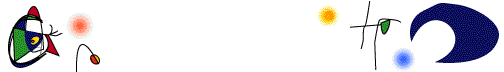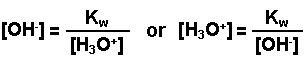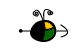The Auto-Ionization of Water, Kw

Water undergoes auto-ionization according to the following equation:

H2O(l) + H2O(l)H3O+(aq) + OH-(aq)
or
2 H2O(l)H3O+(aq) + OH-(aq)

The equilibirum expression for the above reaction is written below and is treated mathematically like all equilibrium expressions.

Kw = [H3O+][OH-]

At 25oC, the value of Kw has been determined to be 1 x 10-14.  This value, because it refers to the auto-ionization of water, has been given a special symbol, Kw, but, it is just a special case of Kc.

If one knows the concentration of either the hydronium ions or of the hydroxide ions in a water solution,. the other ion concentration can be determined.Example:  What is the hydronium ion concentration in a water solution that is 0.050 M NaOH?

The NaOH is a strong base and will 100% dissociate into its component ions.  Therefore, the concentration of the hydroxide ions will be 0.050 M.  The hydronium ion concentration is then calculated.

[H3O+] = (1 x 10-14)/(0.050) = 2.0 x 10-13 M

Example:  What is the hydroxide ion concentration in a water solution that is 4.0 x 10-5 M H3O+?

[OH-] = (1 x 10-14)/(4.0 x 10-5) = 2.5 x 10-10 M